Algebra 1 Worksheets

Basics for Algebra 1 Worksheets

Here is a graphic preview for all of the Basics for Algebra 1 Worksheets. You can select different variables to customize these Basics for Algebra 1 Worksheets for your needs. The Basics for Algebra 1 Worksheets are randomly created and will never repeat so you have an endless supply of quality Basics for Algebra 1 Worksheets to use in the classroom or at home. We have writing variable expressions, order of operations, evaluating expressions, number sets, adding and subtracting rational numbers, multiplying and dividing rational numbers, the distributive property, combining like terms, and percent of change.

Our Basics for Algebra 1 Worksheets are free to download, easy to use, and very flexible.

These Basics for Algebra 1 Worksheets are a good resource for students in the 5th Grade through the 8th Grade.

Click here for a Detailed Description of all the Basics for Algebra 1 Worksheets.

Quick Link for All Basics for Algebra 1 Worksheets

Click the image to be taken to that Basics for Algebra 1 Worksheets.

Writing VariableExpressions Worksheets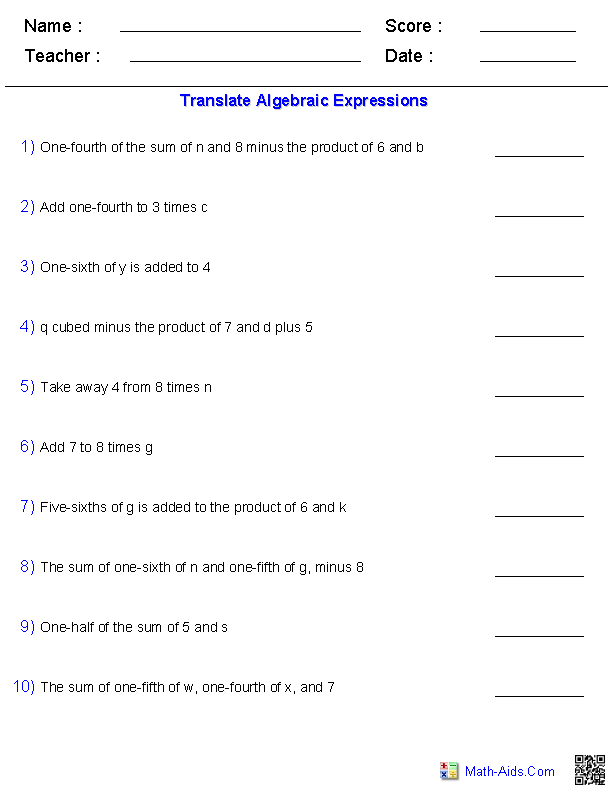Order of OperationsAlgebra 1 Worksheets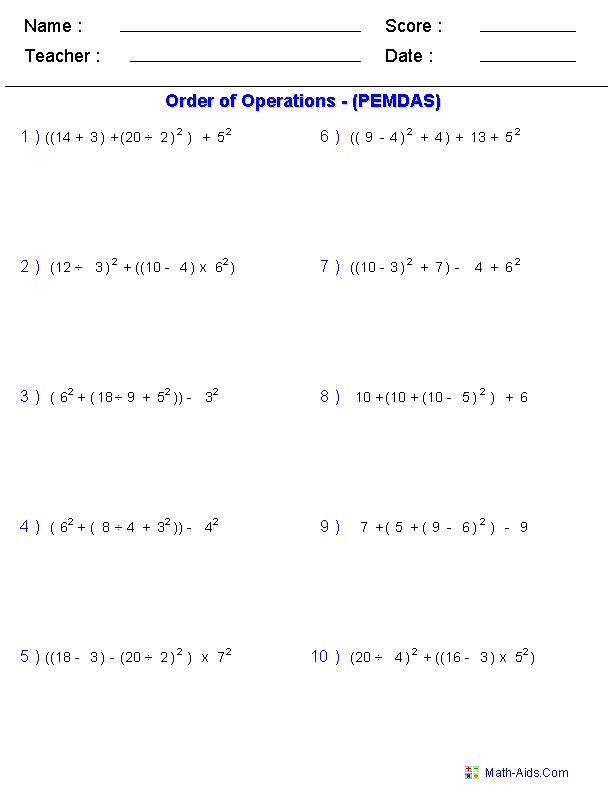Evaluating ExpressionsAlgebra 1 WorksheetsIdentifying Number SetsAlgebra 1 Worksheets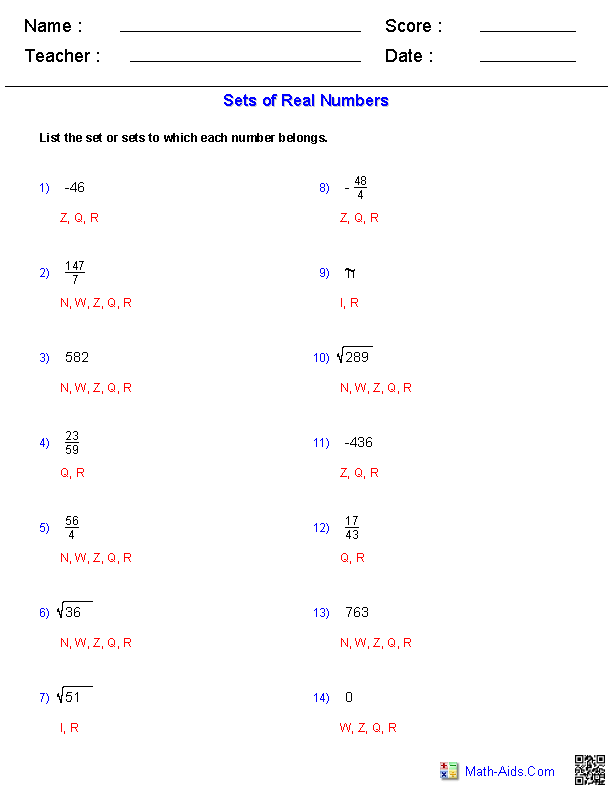Adding and SubtractingRational Numbers Worksheets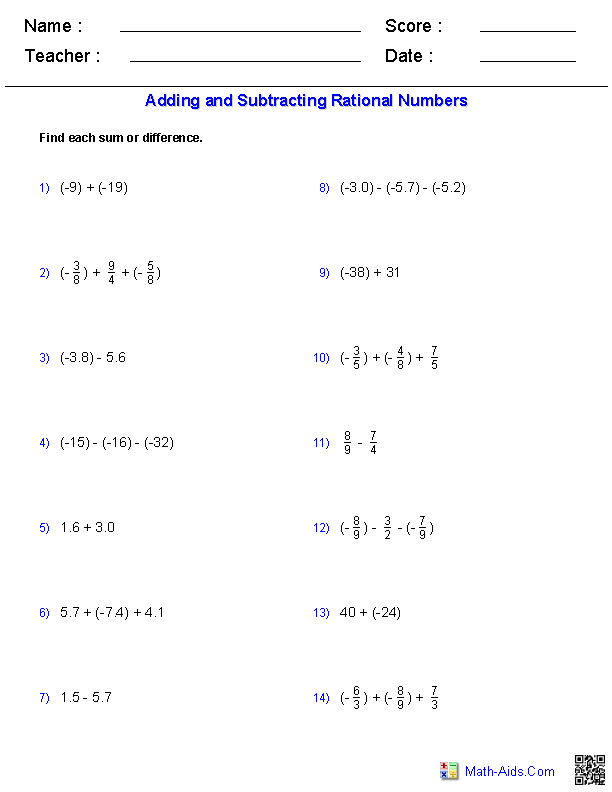Multiplying and Dividing Rational Numbers Worksheets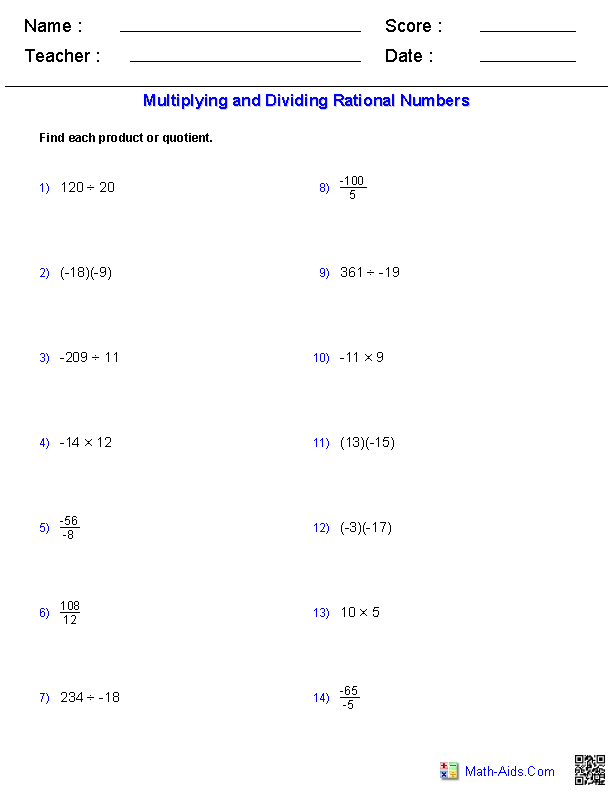The Distributive PropertyAlgebra 1 Worksheets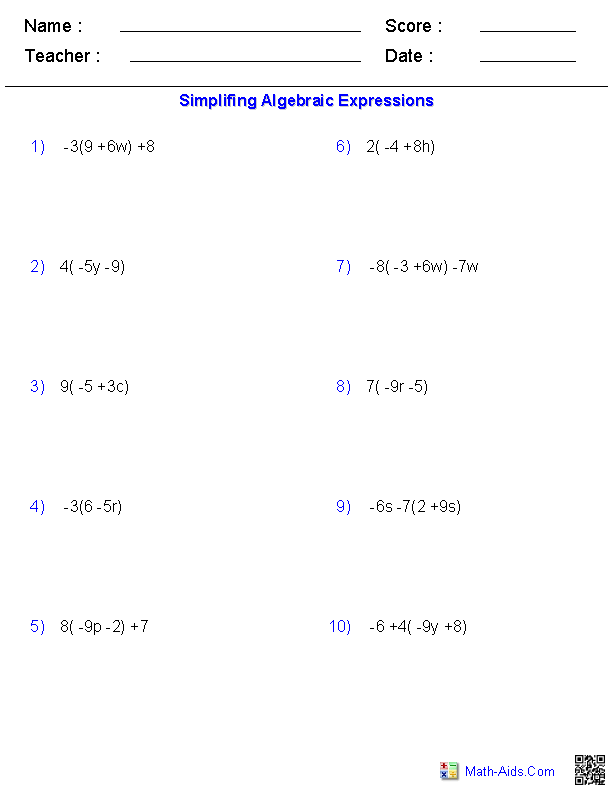Combining Like TermsAlgebra 1 Worksheets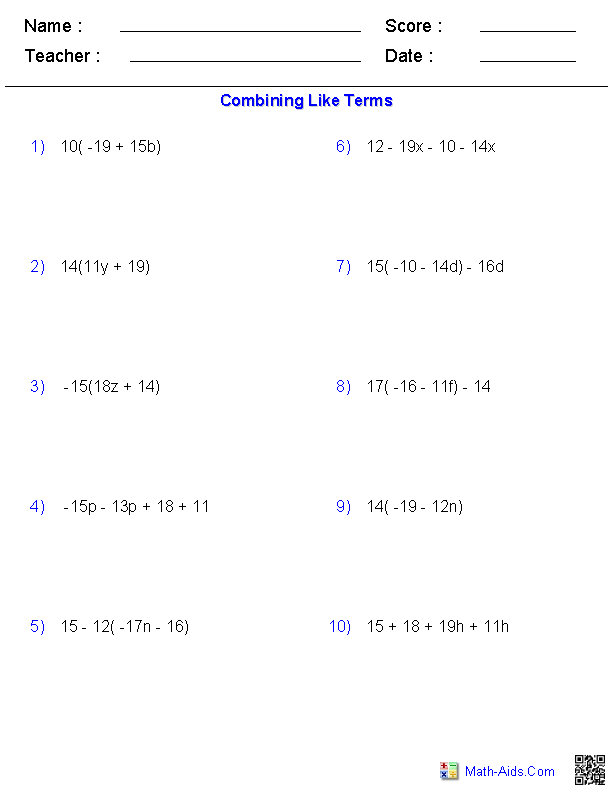Percent of ChangeAlgebra 1 Worksheets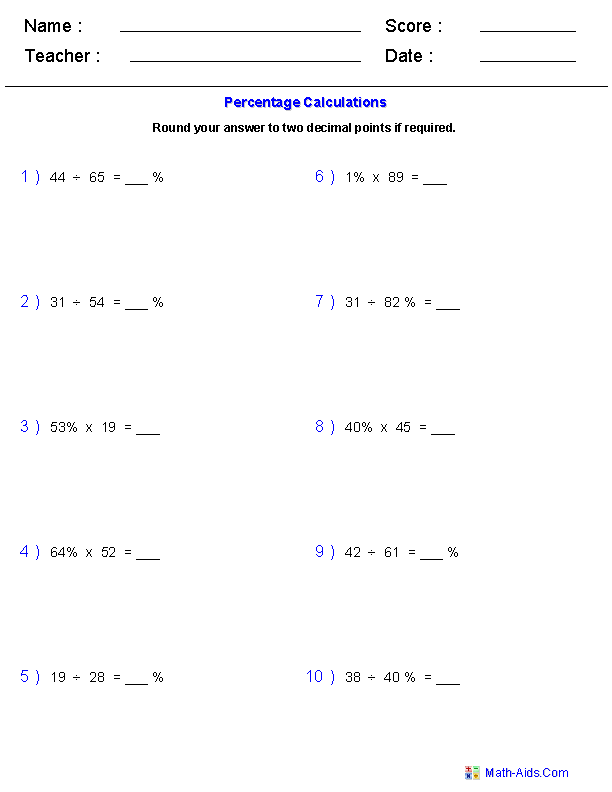Multiply and Divide Decimals and Fractions Algebra 1 Worksheets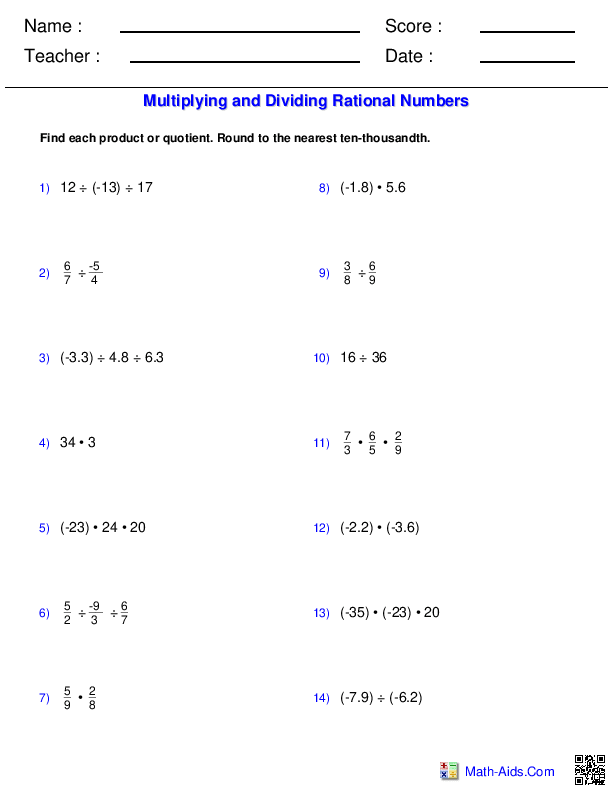Recommended Videos

Detailed Description for All Basics for Algebra 1 Worksheets

Writing Variable Expressions Worksheets
This Algebra 1 - Basics Worksheet will create word problems for the students to translate into an algebraic statements. These basics worksheets will produce ten problems per worksheet. These Basics Worksheets are a good resource for students in the 5th Grade through the 8th Grade.

Order of Operations Worksheets
This Algebra 1 - Basics Worksheet will produce easy or hard problems for practicing Order of Operations calculations. These basics worksheets will produce ten problems per worksheet. These Basics Worksheets are a good resource for students in the 5th Grade through the 8th Grade.

Evaluating Expressions Worksheets
This Algebra 1 - Basics Worksheet will create algebraic statements for the student to evalute. You may select from 2, 3 and 4 terms with addition, subtraction, multiplication, and division. These Basics Worksheets are a good resource for students in the 5th Grade through the 8th Grade.

Identifying Number Sets Worksheets
This Algebra 1 - Basics Worksheet will create problems for the student to identify different number sets. These Basics Worksheets are a good resource for students in the 5th Grade through the 8th Grade.

Adding and Subtracting Rational Numbers Worksheets
This Algebra 1 - Basics Worksheet will create problems for the student to add and subtract rational numbers. These Basics Worksheets are a good resource for students in the 5th Grade through the 8th Grade.

Multiplying and Dividing Rational Numbers Worksheets
This Algebra 1 - Basics Worksheet will create problems for the student to multiply and divide rational numbers. These Basics Worksheets are a good resource for students in the 5th Grade through the 8th Grade.

The Distributive Property Worksheets
This Distributive Property Worksheet will create algebraic statements for the student to simplify. You may select from 3 and 4 terms with addition, subtraction, and multiplication. These Basics Worksheets are a good resource for students in the 5th Grade through the 8th Grade.

Combining Like Terms Worksheets
This Algebra 1 - Basics Worksheets will create algebraic statements for the student to simplify and combine like terms. You may select from 2, 3, or 4 terms with addition, subtraction, and multiplication. These Basics Worksheets are a good resource for students in the 5th Grade through the 8th Grade.

Percent of Change Worksheets
This Algebra 1 - Basics Worksheet is great for practicing percentage calculations. You may select three different types of problems and have the problems be in either numerical or word formats. You may select the range of numbers to work with as well as whole number or decimal numbers. You may specify how many decimal points to round the answers. This percent worksheet will produce ten problems per page. These Basics Worksheets are a good resource for students in the 5th Grade through the 8th Grade.

Multiply and Divide Decimals and Fractions Worksheets
This Algebra 1 - Basics Worksheet will create problems for the student to multiply and divide decimals and fractions. These Basics Worksheets are a good resource for students in the 5th Grade through the 8th Grade.